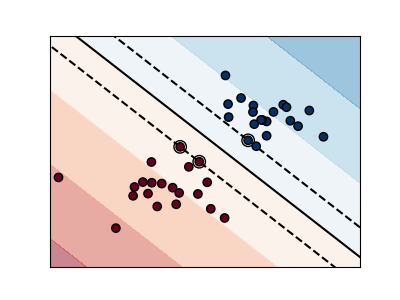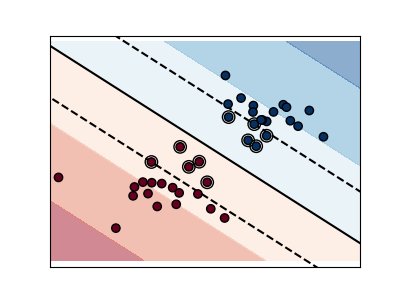# SVM Margins Example¶

The plots below illustrate the effect the parameter C has on the separation line. A large value of C basically tells our model that we do not have that much faith in our data’s distribution, and will only consider points close to line of separation.

A small value of C includes more/all the observations, allowing the margins to be calculated using all the data in the area.

••/home/circleci/project/examples/svm/plot_svm_margin.py:61: UserWarning:

No data for colormapping provided via 'c'. Parameters 'cmap' will be ignored

/home/circleci/project/examples/svm/plot_svm_margin.py:61: UserWarning:

No data for colormapping provided via 'c'. Parameters 'cmap' will be ignored


# Code source: Gaël Varoquaux
# Modified for documentation by Jaques Grobler

import numpy as np
import matplotlib.pyplot as plt
from matplotlib import cm
from sklearn import svm

# we create 40 separable points
np.random.seed(0)
X = np.r_[np.random.randn(20, 2) - [2, 2], np.random.randn(20, 2) + [2, 2]]
Y =  * 20 +  * 20

# figure number
fignum = 1

# fit the model
for name, penalty in (("unreg", 1), ("reg", 0.05)):

clf = svm.SVC(kernel="linear", C=penalty)
clf.fit(X, Y)

# get the separating hyperplane
w = clf.coef_
a = -w / w
xx = np.linspace(-5, 5)
yy = a * xx - (clf.intercept_) / w

# plot the parallels to the separating hyperplane that pass through the
# support vectors (margin away from hyperplane in direction
# perpendicular to hyperplane). This is sqrt(1+a^2) away vertically in
# 2-d.
margin = 1 / np.sqrt(np.sum(clf.coef_**2))
yy_down = yy - np.sqrt(1 + a**2) * margin
yy_up = yy + np.sqrt(1 + a**2) * margin

# plot the line, the points, and the nearest vectors to the plane
plt.figure(fignum, figsize=(4, 3))
plt.clf()
plt.plot(xx, yy, "k-")
plt.plot(xx, yy_down, "k--")
plt.plot(xx, yy_up, "k--")

plt.scatter(
clf.support_vectors_[:, 0],
clf.support_vectors_[:, 1],
s=80,
facecolors="none",
zorder=10,
edgecolors="k",
cmap=cm.get_cmap("RdBu"),
)
plt.scatter(
X[:, 0], X[:, 1], c=Y, zorder=10, cmap=cm.get_cmap("RdBu"), edgecolors="k"
)

plt.axis("tight")
x_min = -4.8
x_max = 4.2
y_min = -6
y_max = 6

YY, XX = np.meshgrid(yy, xx)
xy = np.vstack([XX.ravel(), YY.ravel()]).T
Z = clf.decision_function(xy).reshape(XX.shape)

# Put the result into a contour plot
plt.contourf(XX, YY, Z, cmap=cm.get_cmap("RdBu"), alpha=0.5, linestyles=["-"])

plt.xlim(x_min, x_max)
plt.ylim(y_min, y_max)

plt.xticks(())
plt.yticks(())
fignum = fignum + 1

plt.show()


Total running time of the script: ( 0 minutes 0.081 seconds)

Gallery generated by Sphinx-Gallery